# Input-line-current synthesis in PFC control

Author:
Maurizio Salato, Director of Systems Engineering, Vicor

Date
07/13/2012PDF
##### Digital-control method improves PFC performanceClick image to enlarge

Figure 1: Converter input power vs. line current with a symmetric conduction angle, constant current peak and resistive load.

• CCM (continuous-conduction mode), constant frequency operation enables optimal powertrain design, but operates the converter efficiency curve close to zero at line crossings.
• DCM (discontinuous-conduction mode), variable frequency operation enables better efficiency, but the powertrain and magnetics designs are suboptimal.
• Hybrid approaches such as burst-mode or hysteretic converters present EMC and EMI challenges.
A new technique uses an effective current-modulation angle or dead-band with respect to the line voltage, symmetric around the zero crossing, and based on avoiding the portion of the line cycle not significantly contributing to overall power transfer. Figure 1 shows the relative reduction in power effectively drained from a pure sinusoidal line (resistive load) as a function of a symmetric conduction angle imposed on the input current (orange trace). The blue trace shows that, for example, 15 degrees of electrical angle around the zero crossing are worth just 3% of total available power, but this avoids the lower 25% of the entire line voltage operating range. The impact on the converter is not in avoiding processing the mere 3% of power with lower efficiency, but rather in optimizing powertrain, magnetics, and modulation timing within a narrower voltage range.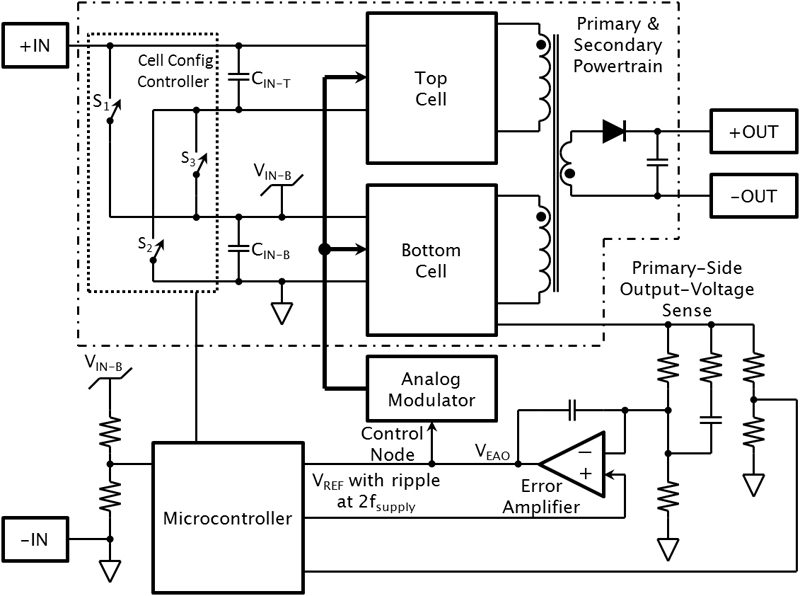Implementation In the PFC block diagram, two primary-stage cells magnetically couple with a single-ended secondary stage, while their inputs are configurable in either series or parallel with respect to the rectified power line (Figure 2). This approach provides several benefits, the most notable being the invariance of efficiency with respect to the input voltage. Controls, which appear at the bottom of Figure 2, consist of a classic analog voltage-regulation loop. This loop manages the control node of an analog modulator to control the converter's output voltage. The microcontroller implements the algorithm: the analog loop reference is actively modulated based on input and output voltage, to achieve power factor correction and shape the input current appropriately. It is important to note that the microcontroller only needs input line frequency and phase information to enable the powertrain outside the dead-band around the line's zero crossing. Although the controller samples the input voltage at a relatively high rate, line frequency acquisition requires few line cycles. This is a minor task for the digital control, resources of which apply to achieve power factor correction and shape the line current. The conduction angle forced on the line current is responsible for 14% of THD. However, the circuit meets all applicable EMC standards. Propagation of line-voltage harmonics Classic PFC relies on direct or indirect measurement of the line voltage, which the controller uses to shape the input current. In cases where the line voltage presents greater harmonic content (typically in heavy industrial environments), the following issue can arise: The current drawn by the input line closely follows the shape of the input line voltage. Harmonics on the voltage waveform is also present in the current waveform, which causes further line drops with the same harmonic content, therefore compounding and worsening the overall power-distribution quality. PFCs often use active compensators in these cases, in order to meet utility regulations, but add operational costs. An alternative approach uses a digital algorithm, with the objective of obtaining a line current shape that meets all applicable IEC standards, achieves high power factor, but does not propagate line voltage harmonics. The technique's targets are:

• To maintain the ratio between input voltage and input current constant, therefore achieving PFC
• To maintain constant the ratio of the input voltage's moving average to the input current's moving average to reduce, and in some cases even cancel, harmonic content in the current waveform.
The introduction of the average term effectively causes a phase delay and affects the power factor by about 1%. The term also significantly reduces line-voltage-distortion propagation to the converter's input current, because higher-order harmonics whose periods falls within the averaging window are reduced over several sampling periods within the modulation algorithm.Equation 1 summarizes how this is achieved: and are the instantaneous values of the converter input voltage and the control node voltage, respectively (as shown in Figure 2), while and are their moving average values. The introduction of the average term effectively causes a phase delay and affects the power factor by about 1%. The term also significantly reduces line-voltage-distortion propagation to the converter's input current, because higher-order harmonics whose periods falls within the averaging window are reduced over several sampling periods within the modulation algorithm.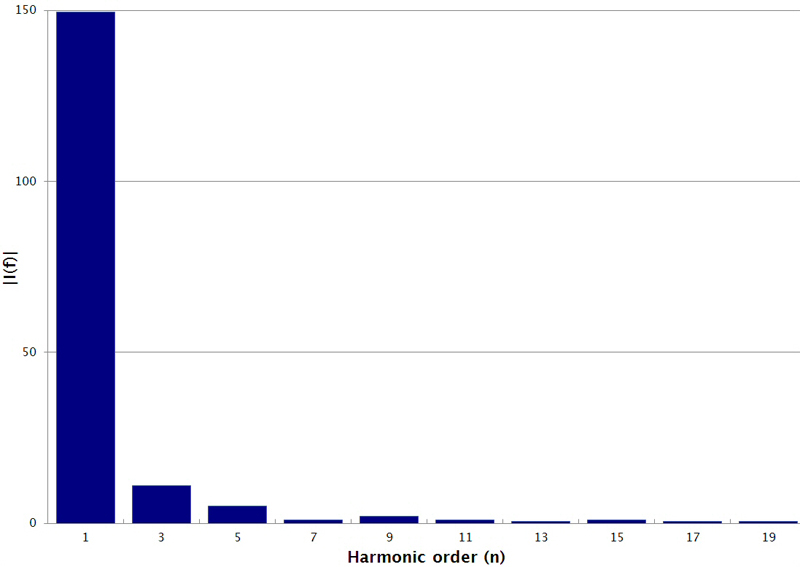Figure 3 shows the harmonic content of a 120 VRMS sinusoid clipped to 80% of its amplitude.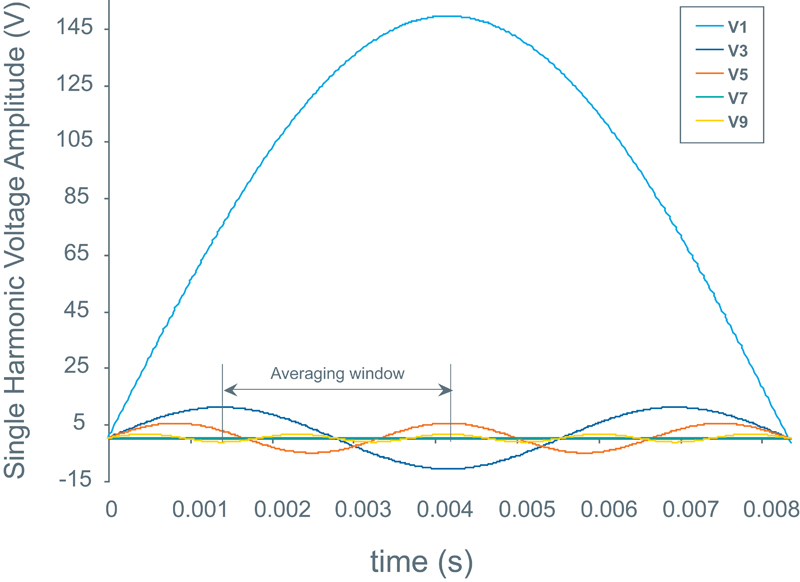Figure 4 shows those harmonics individually in the time domain, with an averaging window for the digital control, which helps to visualize how, by managing sampling rate and window width, higher harmonics are practically canceled. www.vicorpower.com

### RELATED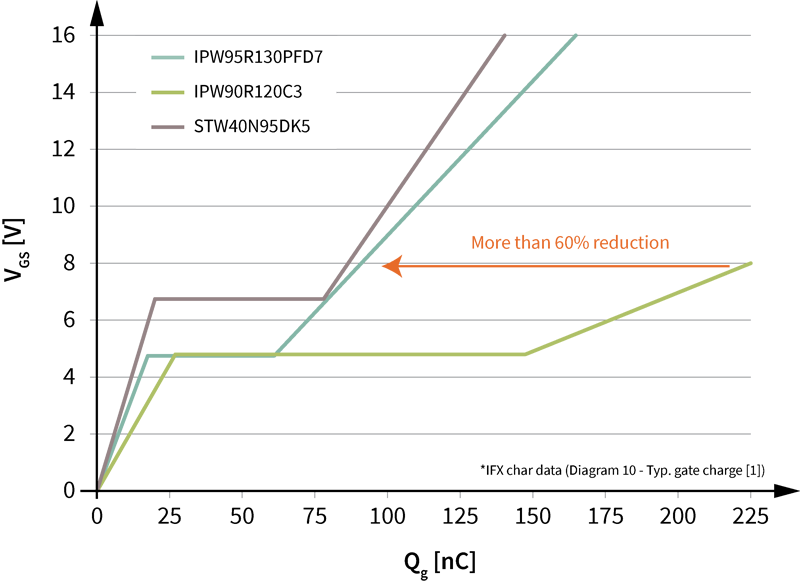#### Ultrahigh power density solutions for energy-efficient high-power lighting and industrial SMPS applications

Jan 23,2023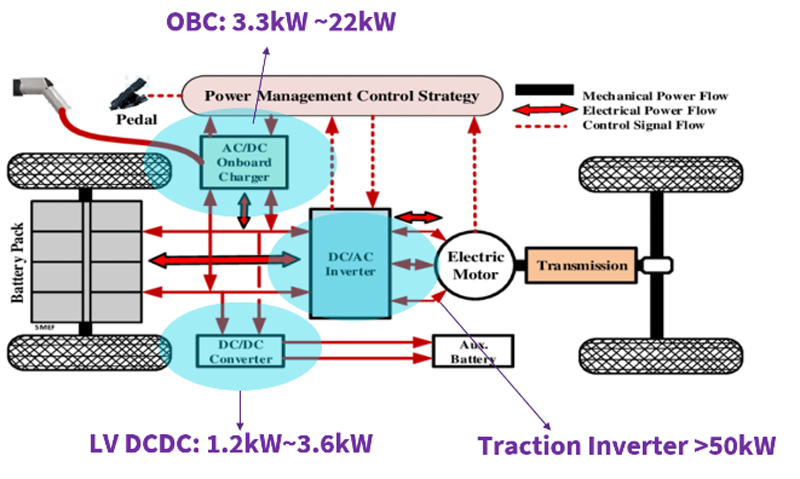#### Wolfspeed Enables Higher-Voltage Batteries for EV Systems

Jan 4,2023#### Power Modules Accelerate the Path to Long-Haul, Autonomous Trucking

Dec 31,2022Jan 1,2023